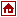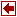MPL
Mathematical Programming Language
 Author: Maximal Software, Inc. 2111 Wilson Boulevard  Suite 700  Arlington, VA 22201  U.S.A.

Structure of the MPL Model File

The MPL model file is divided into two main parts; the definition and the model. In the definition part you define various items that are then used throughout the model. The model part, on the other hand, contains the actual model formulation. Each part is further divided into sections, which are as follows:

The Definition Part

• TITLE - The problem name
• INDEX - The dimension of the problem
• DATA - Scalars, datavectors and datafiles
• DECISION - Define vector variables
• MACRO - Reusable macros for expressions
The Model Part
• MODEL - Description of the problem
• MAX or MIN - The objective function
• SUBJECT TO - The constraints
• BOUNDS - Simple upper and lower bounds
• INTEGER - Integer variables
• BINARY - Binary (0/1) variables
• FREE - Free variables
• END
The objective function and the constraints are required; all other sections are optional. Either of the keywords MAX or MIN in the objective function is required; the others are optional. The keywords TITLE, INDEX, DATA, DECISION VARIABLES, MACRO, BOUNDS, FREE, INTEGER, and BINARY are used only when the model contains their respective sections. The keywords MODEL, SUBJECT TO and END are used to aid readability.

Sets and Indexes

In the index section you define the domains of the problem.The indexes are then used throughout the model when objects like subscripted variables and data coefficients, summations and structured constraints are used. Indexes encapsulate the problem dimensions and make it easy to quickly adjust the problem size. There are two basic types of indexes; integer and named. The integer indexes are used to give each subscript item a numerical value. The named indexes are used to give each subscript item a descriptive name.

Set Operations on Indexes

When working with subsets of indexes, you sometimes want to create new indexes based on previous ones. MPL allows you to use standard set operations such as difference, not, union, and intersection to help define new indexes.

Data For The Model

In the data section you specify the data coefficients to be used in the model. These can be scalars, vectors, and even arrays of multiple dimensions. By defining the data coefficients separately, the actual model is free of numerical data and thus easier to maintain. Furthermore, by using the import feature you can store the data in a separate file and retrieve it when generating the input file. Each data coefficient is given a separate name that is used throughout the model. There are two different types of data objects that MPL recognizes; data constants and data vectors.

Database Connection

MPL offers as an option, the capability to import indexes and data, that is needed for the model, directly from a database. After optimizing the problem, you can then also export the solution values for the variables and the constraints back to the database.

Conditional Directives

`This feature in MPL is well known in computer programming. Conditional directives can be just as useful when developing linear programming models as when developing programs. They allow you to define special symbols called directives and then, based on these directives, to include or exclude some part of the model.

References